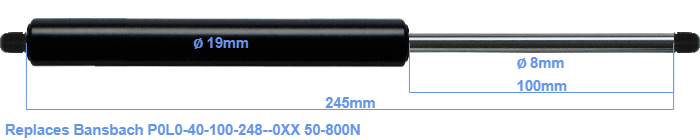# Replacement for Bansbach P0L0-40-100-248–0XX 50-800N

\$28.57

Replacement gas spring for the Bansbach P0L0-40-100-248--0XX 50-800 Newton. Thread: M8. Brand: Stabilus Industry Line.
 Force Choose an option50 Newton60 Newton80 Newton100 Newton120 Newton140 Newton150 Newton160 Newton180 Newton200 Newton220 Newton240 Newton250 Newton260 Newton280 Newton300 Newton320 Newton340 Newton350 Newton360 Newton380 Newton400 Newton420 Newton440 Newton450 Newton460 Newton480 Newton500 Newton520 Newton540 Newton550 Newton560 Newton580 Newton600 Newton620 Newton640 Newton650 Newton660 Newton680 Newton700 Newton720 Newton740 Newton750 Newton760 Newton780 Newton800 NewtonClearThe diameter of the black cylinder of this gas spring is 19 millimeter. The diameter of the rod is 8 millimeter. The rod (the chrome part) is 100 mm long. The total length of this replacement is exactly 245 mm. Note: this is the total length between the rotation points of the two mounting parts. The length from thread to thread (wihout mounting parts) is 245 mm. This gas spring (P0L0-40-100-248--0XX) has a force of 50-800 Newton. This is not an original gas spring produced by Bansbach, but it can replace a Bansbach gas spring. This a gas spring with the brand Stabilus Industry Line. The gas spring can be used without problems as a replacement, because the dimensions and force equal.